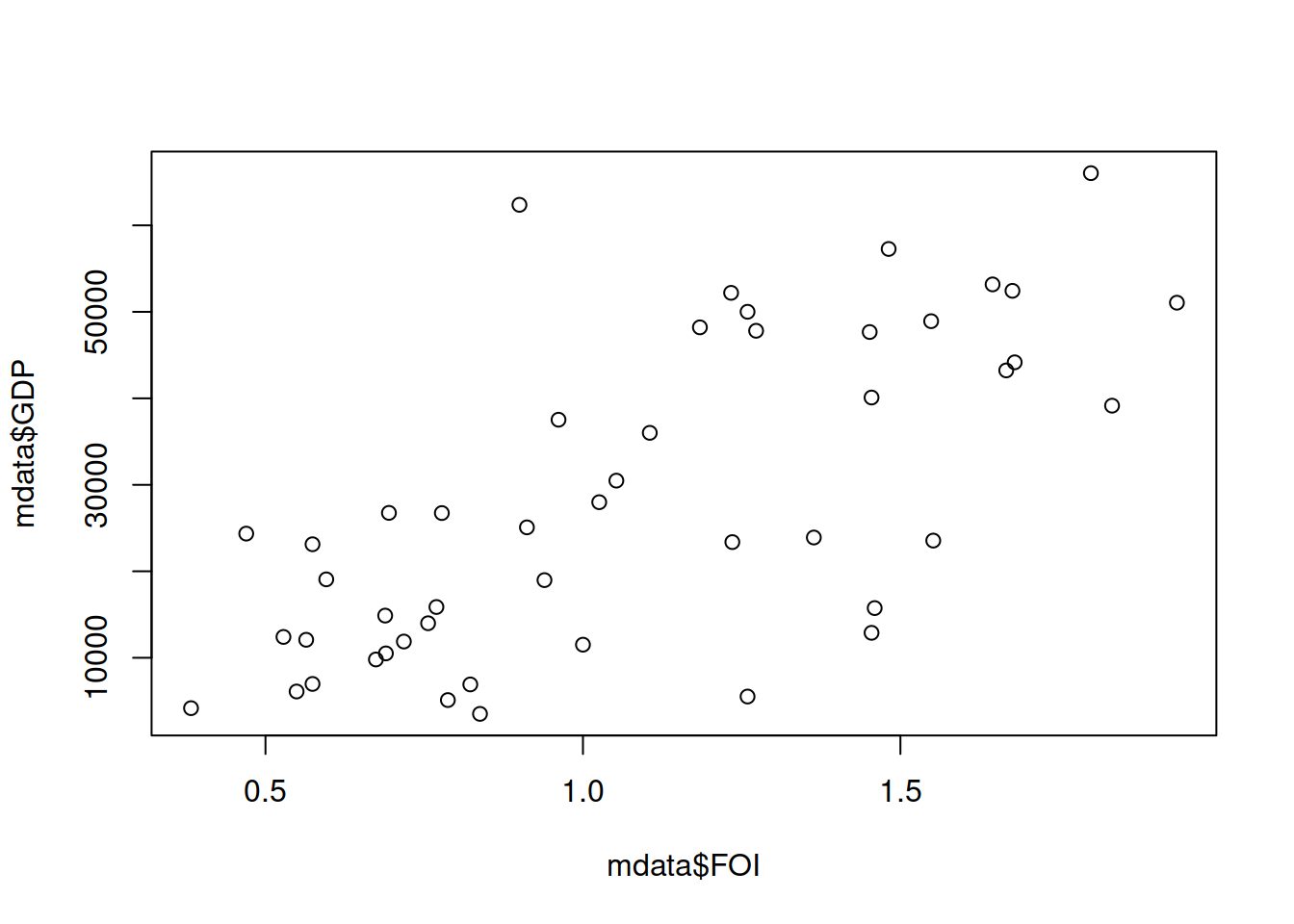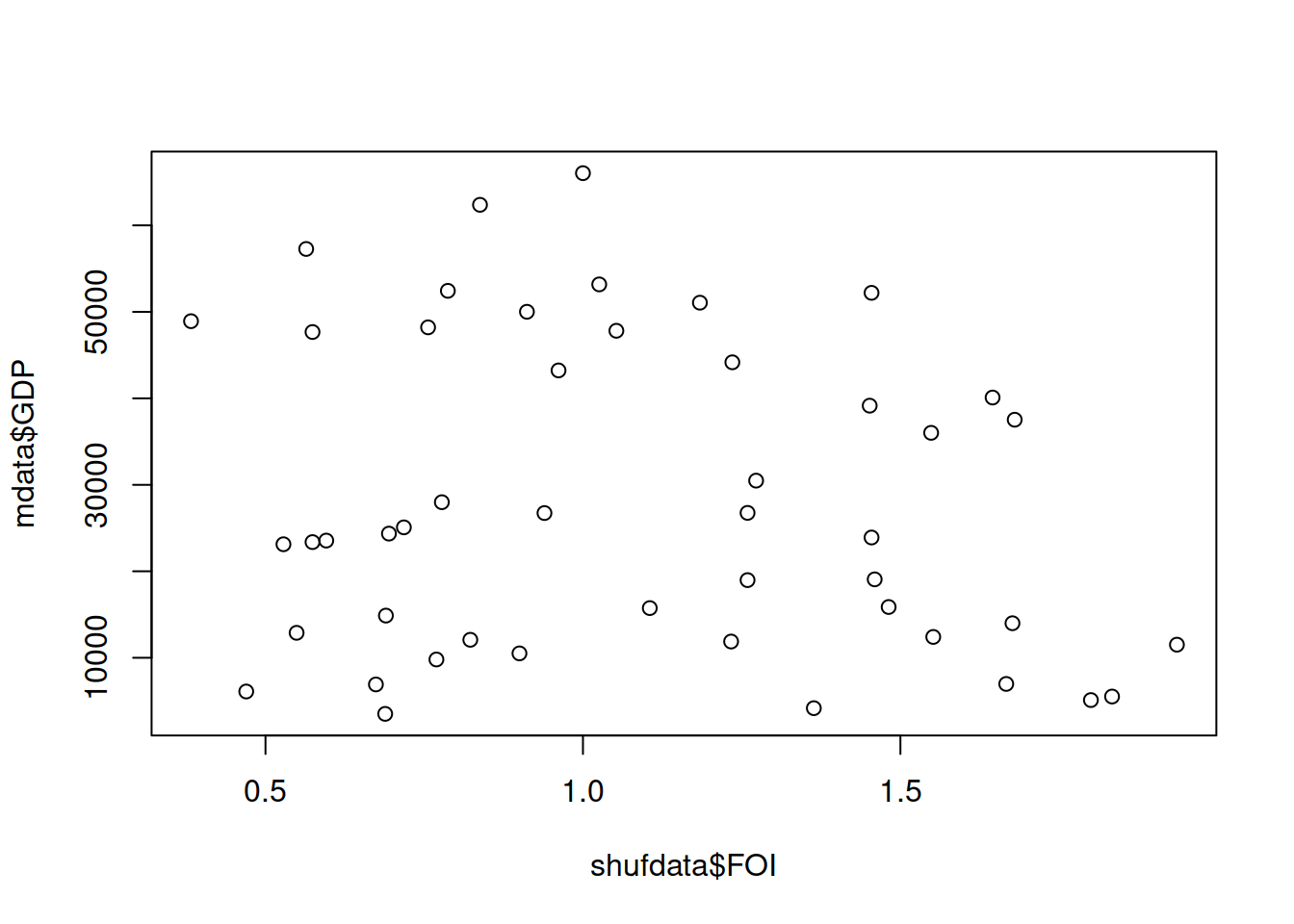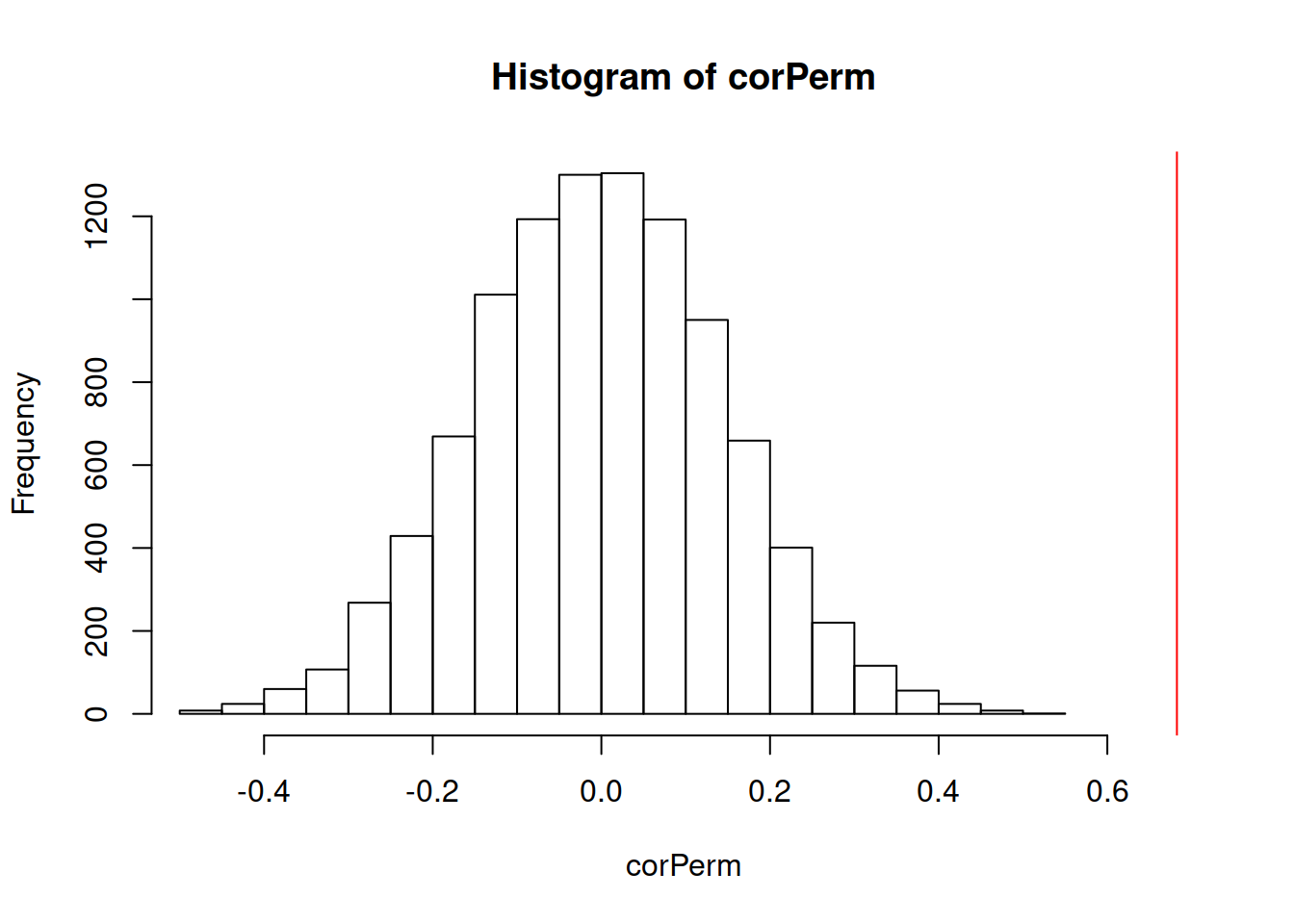### Permutation tests

Once we have measured something or got a statistical result from our analysis, we might ask ourselves: What is the probability that this result can appear due to chance alone? For example, imagine that we measure the correlation coefficient between two variables $$X$$ and $$Y$$: $$\rho(X,Y)$$. We should ask ourselves what is the probability that we could measure a similar or even stronger correlation if $$X$$ and $$Y$$ were completely independent. Since our sample sizes are finite, there is always a chance that we measure some nonzero correlation, thus we need an idea of how plausible is this measurement in a situation in which there is no real association between variables.

We can answer this questions with a permutation test. A permutation test has four parts:

1. A test statistic, for example in this case the correlation coefficient between $$X$$ and $$Y$$: $$\rho(X,Y)$$
2. A null hypothesis, which is a statement about the value of our test statistic when “nothing interesting happens”. It is often denoted as $$H_0$$ and in this case it would be $$H_0: \rho(X,Y)=0$$.
3. An alternative hypothesis, which is another statement about the value of the test statistic that stems from our theory or research question. It is often denoted as $$H_1$$ and, for example, it could be $$H_1: \rho(X,Y)>0$$. The alternative hypothesis and the null hypothesis have to be contradictory, i.e. both can’t be true at the same time, but both could be false at the same time.
4. A permutation set with $$N$$ random permutations or shuffles of the data. Permutations in this set model a world in which the null hypothesis is true and any measurement different than the one specified in the null hypothesis is due to chance alone. $$N$$ should be relatively large so we have an idea of the range of values of the test statistic when it is produced only by chance.

### Permutation tests in R

At the end of the GDP and FOI exercise, we permuted the FOI vector and calculated the correlation coefficient. Before the permutation, the scatter plot and correlation between GDP and FOI looked like this:

plot(mdata$FOI, mdata$GDP)cor(mdata$FOI, mdata$GDP)
##  0.6825286

And after the permutation, they looked like this:

shufdata <- mdata[sample(nrow(mdata)),]
plot(shufdata$FOI, mdata$GDP)cor(shufdata$FOI, mdata$GDP)
##  -0.1857091

Before the permutation, the correlation coefficient was well above zero, but afterwards it was very close to zero. A permutation test repeats this process many times and compares the distribution of values of the correlation coefficient (our test statistic) in the permutations with the value we measured in the original dataset. Usually we want to calculate several thousands of permutations, which is a task that was computationally expensive years ago but that is very easy and quick to do for modern computers.

We can do the permutation test in R with a loop like this:

N <- 10000 # repeat shuffling for N times
corPerm <- numeric(length = N) # vector with the results of the test statistic under each permutation
for(i in 1:N)
{
shufdata <- mdata[sample(nrow(mdata)),]
corPerm[i] <- cor(shufdata$FOI, mdata$GDP)
}
corObserved <- cor(mdata$FOI, mdata$GDP)

After that, we have the values of the correlation coefficient in the permuted samples in corPerm and the empirical value in our dataset in corObserved. We can compare them by plotting a histogram of the permuted values and a line for the value in our dataset:

hist(corPerm, xlim=range(c(corPerm,corObserved)))
abline(v=corObserved, col="red")When the empirical value is very far from the permutation results, like in the case above, we can be pretty sure that a correlation coefficient as strong as the one we measured is unlikely to appear in a situation of pure chance when the variables are truly independent.

### The p-value of a permutation test

The p-value is a way to summarize the results of a permutation test.

p-value: Given that the null hypothesis is true, the p-value is the probability that we measure a statistic at least as extreme as the observed result

p-values are often misused and misunderstood and you might have heard about it before. A common misconception is that the p-value measures the probability that the null hypothesis is true. The p-value measures the plausibility of what we measure under the null hypothesis, which is very different.

When our alternative hypothesis is a statement about the test statistic being positive or negative, we calculate a one-sided p-value as the proportion of permutations with a value of the statistic at least as large as the observed one. In case our alternative hypothesis says that the test statistic is different than zero, we calculate a two-sided p-value as the proportion of permutations with an absolute value of the statistic at least as large as the observed one.

We can calculate the two-sided p-value from the permutation test on the previous example:

p_value_Cor <- (sum(corPerm>=corObserved)+1)/length(corPerm)
p_value_Cor
##  1e-04

Above, we measure the area under the histogram of permutation values above the empirical one as our estimation of the p-value. We add 1 to the numerator to give an upper bound to p, so it is never zero because there is always some chance that our test statistic can be measured for some permutation. If you look at the histogram we plotted earlier, there wasn’t even one case of a permutation with a correlation above the observed one, hence the very low p-value.

A common threshold to argue about p-values is 0.05. Many scientific communities call this a “significant” result, but the term is rather confusing because it does not mean anything about the importance of the result. If you want to be confident that your measured p is below 0.05, it is recommended to permute at least $$10000$$ times so that you have enough permutations. When running some R package for statistical analysis, you will often get some p-value results in the output. Before coming to conclusions, it is important that you read the documentation to find out how it has been calculated and what are the null and alternative hypotheses. In case of doubt, it never hurts to do your own permutation tests.

Permutations here are just an example of what is called a null model. You can do the same as you did here for any null model to understand the plausibility of your empirical measurement in a random model that keeps some properties as your original data, for example correlations between independent variables in a regression model or even network properties in a random network model.